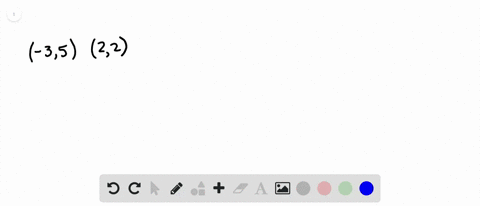Enroll in one of our FREE online STEM summer camps. Space is limited so join now!View Summer Courses### Write an equation in slope-intercept form for the…

01:50
AG

Need more help? Fill out this quick form to get professional live tutoring.

Get live tutoring
Problem 3

Write an equation in slope-intercept form for the line that satisfies each set of conditions.
slope $3,$ passes through $(0,-6)$

$y=3 x-6$

## Discussion

You must be signed in to discuss.

## Video Transcript

the given question is right and equation and slow intercept form for the line that satisfy each sort of condition. And the sort of condition given is so is three, and the line passes through the 30.0 comma minus six. We know the general equation off line in slope intercept form is why is equal to mm x plus C Here M is the slow off the line and see is the white and disip huge From the given condition, it can be observed that the value of C is minus six and the slope is three. Substitute the values in the general form toe pain. The equation bye is equal to three x bless, minus six that simplifies to give by his acquittal three x minus six, which is there?